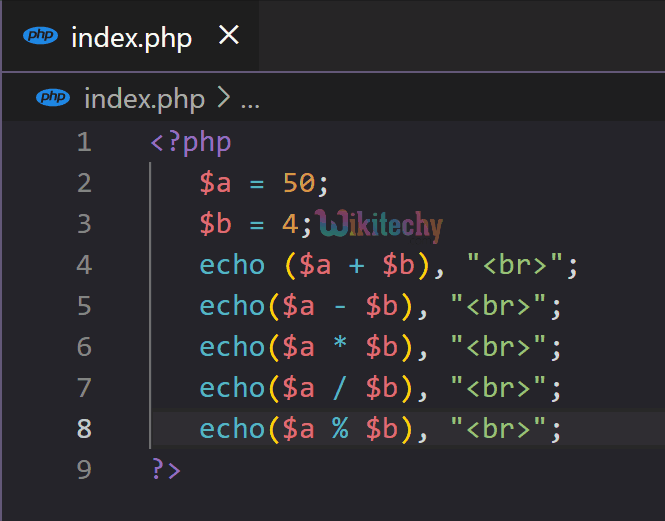# PHP Arithmetic Operators - Arithmetic Operators in PHP

## PHP Arithmetic Operators

• PHP arithmetic operators are used to perform various operations on numeric operands.
• These operations include addition, subtraction, multiplication, division, and modulus. The basic arithmetic operators are +,-,*,/, and %.
Operator Syntax Operation
Addition ( + ) \$a + \$b Sum the operands
Subtraction ( - ) \$a – \$b Differences the operands
Multiplication ( * ) \$a * \$b Product of the operands
Division ( / ) \$a / \$b The quotient of the operands
Exponentiation ( ** ) \$a ** \$b \$a raised to the power \$b
Modulus ( % ) \$ a% \$b The remainder of the operands